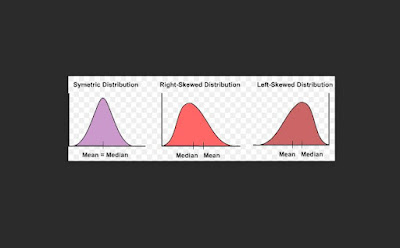## Sunday, March 28, 2021

### Skewed DistributionsSkewed Distributions*

Skewed distributions have one tail that is longer than the other tail compared to the "normal" distribution, which is perfectly symmetrical. Skew affects the location of the central values of the mean and median.

Positive Skew

Below is an image of positive skew, which is also called right skew. Skew is named for the "tail." If you had statistics, you may have heard a professor say, "the tail tells the tale." The tail is the extended part of the distribution close to the horizontal axis.

The large "hump" area to the left represents the location of most data. In behavioural science, the high part often refers to the location of most of the scores. Thus, in positively skewed distributions, most of the participants earned low scores and few obtained high scores as you can see by the low level of the curve, or the tail, to the right.

Negative Skew

As you might expect, negatively skewed distributions have the long tail on the left thus, they are also called left-skewed distributions. A negatively skewed distribution of test scores illustrates an easy test--just what students want. Teachers used to talk about grading on a curve. You can see that such grading could be good or bad for students depending on what curve the teacher uses.

Skewed distributions are nonnormal by definition.

Recall that in the normal curve, the mean, median, and mode are all at the same point in the middle of the distribution. The value of skew in a normal distribution is zero.

In skewed distributions, the mode is at the high point and it represents the most frequent value or test score. The mean is pulled in the direction of the long tail and the median falls between the mode and the mean.

Common test questions ask what happens to the mean in skewed distributions. Keep in mind that the mean is "pulled" toward the tail. The mean is an average and, as such, it is most susceptible to extreme scores.

Skew and Data Analysis

Most statisticians accept small deviations from normality when analysing data using procedures designed for a normal distribution like the Pearson r, t tests, and the parametric ANOVAs

The question of acceptable ranges of skew will yield different answers from different sources. A range of +1.5 to -1.5 is a common rule of thumb. An important consideration is the "true" nature of the measured variable. Scientists may argue for flexibility in analysing data from a sample if the variable is known to be normally distributed in the population.

Skewed data can be adjusted and should be adjusted before using parametric tests. One method of adjustment is to convert all scores to logarithms and perform the data analysis on these transformed values.

If the data are too skewed and it is inappropriate to transform the data, then analysts should use nonparametric statistical methods.

Moments

In statistics, the concept of moments is taken from physics. Moments refer to central values. The first moment is found by calculating the value of the mean. The first moment is zero.

The second moment is seen in the calculation of variance, which uses squared values.

The third moment is found by calculating skew and the fourth moment results in the calculation for kurtosis.

and see my books on

Also, consider connecting with me on    FACEBOOK

You can read many published articles at no charge:

Academia   Geoff W Sutton     ResearchGate   Geoffrey W Sutton

*Photo credit- From Bing images labeled "Free to share and use."